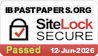# Topic 8: Energy production

## 8.1 – Energy sources

• Specific energy and energy density of fuel sources
• Specific energy and energy density are useful measures of the energy that will be released from a given weight or volume of fuel when it is burned.
• Specific energy is the energy per unit mass of the fuel and can be given by energy/mass
• Energy density is the energy per unit volume of a fuel and can be given by energy/volume.
• Sankey diagrams

• While energy may be completely converted into work in a single process, a cyclical process is required to convert thermal energy to work continuously.
• In accordance with the second law of thermodynamics, some energy will be lost to the surroundings and cannot be used to perform useful work. The unavailable energy is called degraded energy.

Sankey diagram

• A Sankey diagram is a type of energy diagram where the width of each arrow is proportional to the amount of energy in that section.
• Primary energy sources

Primary energy sources are found in nature and have not been subjected to any conversion or transformation process.

• Electricity as a secondary and versatile form of energy

Electricity is a secondary and versatile form of energy.

Production of electrical power:

1. Fuel is used to boil water to produce pressurized steam.
2. The pressurized steam drives a turbine which is connected to coils of wire.
3. When the turbine rotates, the coils of wire move through a strong magnetic field. Electrical power is produced via electromagnetic induction in the generator.
• Renewable and non-renewable energy sources
• There are two categories of primary energy sources: renewable energy resources and non-renewable energy resources.
• Renewable energy sources are the ones which cannot be depleted.
• Non-renewable energy sources can be used up and become depleted.
• Most energy sources derive their energy from the sun directly (e.g. solar power) or indirectly (e.g. fossil fuels).

## 8.2 – Thermal energy transfer

• Conduction, convection and thermal radiation

Conduction and convection requires a transmittance medium while radiation does not and can be transferred through a vacuum (e.g. outer space).

• A black body which absorbs all incident electromagnetic radiation is both the perfect absorber and the perfect emitter of radiation.
• The radiation emitted by such a body at constant temperature is called black-body radiation.
• The Stefan-Boltzmann law states that the power of radiation emitted by a black body per unit area is proportional to the fourth power of its temperature.

Emission spectrum of a black body

• Albedo and emissivity

Albedo

• The Albedo (α) of a planet is defined as the ratio between the total scattered (reflected) radiation and the total incident radiation of that planet.
• Albedo = total scattered power / total incident power
• The albedo of a planet is affected by the following:
1. Season (cloud formations)
2. Latitude
3. Terrain (ocean has low albedo because it mainly absorbs and snow has high albedo because it mainly reflects)
• The global annual mean albedo is about 0.3 (30%) on Earth.

Emissivity

• Most objects are not black bodies. They radiate a fraction of the power per unit area compared to a black body at the same temperature. The value of this fraction depends on the object and is called the object’s emissivity (e).
• Emissivity = power per unit area radiated by the object / power per unit area radiated by a black body at the same temperature.
• The equation for the power radiated by an object with emissivity e can be given by the diagram in the previous section (Black-body radiation).
• The solar constant
• The solar constant is the amount of energy that normally falls on a unit area (1m^2) of the Earth’s atmosphere per second when the Earth is at its mean distance from the sun.
• The solar constant is approximately 1366 W/m^2.
• The greenhouse effect

Greenhouse gases:

• Greenhouse gases absorb infrared radiation because its molecules have natural frequencies in the infrared region and readily absorb infrared radiation due to resonance.

Mechanism:

1. Incoming radiation from the sun takes form of ultraviolet and visible radiation.
2. Some of this radiation is absorbed by the Earth’s atmosphere and some of the radiation is reflected back into space by the Earth’s surface (ground).
3. The radiation which is reflected back into space takes form of infrared radiation.
4. The greenhouse gases present in the atmosphere absorb infrared radiation and reflect it back towards the Earth’s surface.
5. Thus, heat energy becomes trapped inside Earth’s atmosphere and accumulates, leading to the greenhouse effect and an increase in average mean temperatures on Earth.

Chain reaction:

• Global warming reduces ice/snow cover. This causes a decrease in albedo and increases the rate of heat absorption by the Earth.
• The solubility of carbon dioxide in the sea decreases with increasing temperature, leading to an increase in atmosphere carbon dioxide (greenhouse gas) concentration.

Effects:

• Rise in mean sea level by the melting of ice on land.
• Extreme weather such as heat waves and heavy floods.
• Energy balance in the Earth surface–atmosphere system

Earth’s energy balance describes how the incoming energy from the sun is used and returned to space. If incoming and outgoing energy are in balance, the Earth’s temperature remains constant.

Topic 8: Energy production